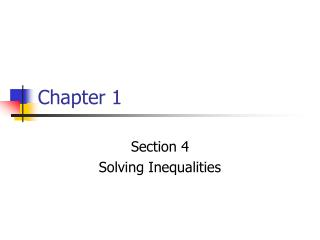DownloadDownload PresentationChapter 1

# Chapter 1

Download Presentation## Chapter 1

- - - - - - - - - - - - - - - - - - - - - - - - - - - E N D - - - - - - - - - - - - - - - - - - - - - - - - - - -
##### Presentation Transcript

1. Chapter 1 Section 4 Solving Inequalities

2. Solving Inequalities < < – – > > – – 7. 3x + 3 = 2x – 3 1. 5 < 12 8. 5x = 9(x – 8) + 12 2. 5 < –12 3. 5 12 4. 5 –12 5. 5 5 6. 5 5 ALGEBRA 2 LESSON 1-4 (For help, go to Lessons 1-1 and 1-3.) State whether each inequality is true or false. Solve each equation.

3. Solving Inequalities < < – – > > – – ALGEBRA 2 LESSON 1-4 Solutions 1. 5 < 12, true 3. 5 12, false 5. 5 5, true 7. 3x + 3 = 2x – 3 3x – 2x = –3 – 3x = –6 2. 5 < –12, false 4. 5 –12, false 6. 5 5, true 8. 5x = 9(x – 8) + 12 5x = 9x – 72 + 12–4x = –60x = 15

4. Inequalities • The solutions include more than one number • Ex: 2 < x ;values that x could be include 3, 7, 45… • All of the rules for solving equations apply to inequalities, with one added: • If you multiply or divide by a NEGATIVE you must FLIP the sign. (< becomes > and > becomes <) • When graphing on a number line: • Open dot for < or > • Closed (solid) dot for ≤ or ≥ • The shading should be easy to see (a slightly elevated line is ok) --- see examples

5. Solving Inequalities ALGEBRA 2 LESSON 1-4 Solve –2x < 3(x – 5). Graph the solution. –2x < 3(x – 5) –2x < 3x – 15 Distributive Property –5x < –15 Subtract 3x from both sides. x > 3 Divide each side by –5 and reverse the inequality.

6. Try These Problems Solve each inequality. Graph the solution. • 3x – 6 < 27 • 3x < 33 x < 11 • 12 ≥ 2(3n + 1) + 22 • 12 ≥ 6n + 2 + 2212 ≥ 6n + 24-12 ≥ 6n-2 ≥ n

7. 0 Solve 7x≥ 7(2 + x). Graph the solution. 7x≥ 7(2 + x) 7x≥ 14 + 7x Distributive Property 0 ≥ 14Subtract 7x from both sides. The last inequality is always false, so 7x≥ 7(2 + x) is always false. It has no solution.

8. 0 0 Try These Problems Solve. Graph the solution. • 2x < 2(x + 1) + 3 • 2x < 2x + 2 + 32x < 2x + 5 0 < 5All Real Numbers • 4(x – 3) + 7 ≥ 4x + 1 • 4x – 12 + 7 ≥ 4x + 1 4x + 12 ≥ 4x + 1 12 ≥ 1 No Solution

9. > > > > – – – – Relate: \$2000 + 4% of sales \$5000 Define: Let x = sales (in dollars). Write: 2000 + 0.04x 5000 0.04x 3000 Subtract 2000 from each side. x 75,000 Divide each side by 0.04. Solving Inequalities A real estate agent earns a salary of \$2000 per month plus 4% of the sales. What must the sales be if the salesperson is to have a monthly income of at least \$5000? The sales must be greater than or equal to \$75,000.

10. Try This Problem A salesperson earns a salary of \$700 per month plus 2% of the sales. What must the sales be if the salesperson is to have a monthly income of at least \$1800? 700 + .02x ≥ 1800 .02x ≥ 1100 x ≥ 55000 The sales must be at least \$55,000.

11. Compound Inequalities • Compound Inequality – a pair of inequalities joined by and or or • Ex: -1 < x and x ≤ 3 which can be written as -1 < x ≤ 3 • x < -1 or x ≥ 3 • For and statements the value must satisfy both inequalities • For or statements the value must satisfy one of the inequalities

12. And Inequalities • Graph the solution of 3x – 1 > -28 and 2x + 7 < 19. 3x > -27 and 2x < 12 x > -9 and x < 6 • Graph the solution of -8 < 3x + 1 <19 -9 < 3x < 18 -3 < x < 6

13. Or Inequalities 3x < –12 –2x < 4 ALGEBRA 2 LESSON 1-4 Graph the solution of 3x + 9 < –3 or –2x + 1 < 5. 3x + 9 < –3 or –2x + 1 < 5 x < –4 or x > –2

14. Try These Problems • Graph the solution of 2x > x + 6 and x – 7 < 2 • x > 6 and x < 9 • Graph the solution of x – 1 < 3 or x + 3 > 8 • x < 4 or x > 11

15. Homework • Practice 1.4 All omit 23